# ML-集成学习：AdaBoost、Bagging、随机森林、Stacking(mlxtend)、GBDT、XGBoost、LightGBM、CatBoost原理推导及实现

1.名称简介

2.设计集成原则

3.集成学习算法分类

4.Boosting

4.1基本流程

4.2Adaboost实现

4.2.1.分类

4.2.2.回归

4.2.3Adaboost的正则化

4.2.4Adaboost小结

5.Bagging

5.1基本流程

6.随机深林Random Forest

6.1基本流程

7.Stacking

8.GBDT

8.1 GBDT回归

8.2GBDT分类

9.XGBoost

1）定义结构函数q(x)

2）输出函数的叠加形式为：

3）第 t 轮的目标（损失）函数为：

4）结构评价函数

5）确定最佳分裂准则

6）分裂流程

10.LightGBM

11.CatBoost

# 1.名称简介# 2.设计集成原则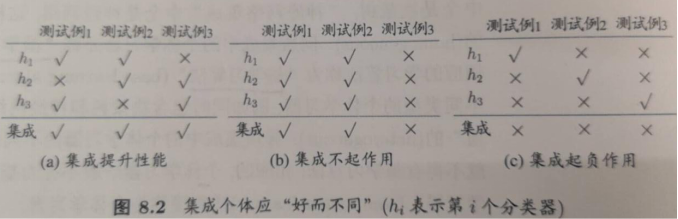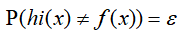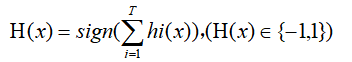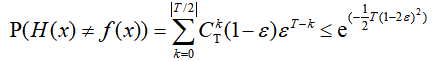# 3.集成学习算法分类

1）个体学习器有强依赖，必须串行生成序列方法：Boosting(AdaBoost、GDBT、XGBoost)

2）不存在强依赖，可同时生成的并行方法：Bagging和 随机森林（Random Forest）

# 4.Boosting

## 4.1基本流程## 4.2Adaboost实现

Adaboost既可以用作分类，也可以用作回归。

### 4.2.1.分类

CART的优点就是可以分类和回归，这和AdaBoost一样，因此很适合做为其基础学习器，实际上，后面使用的sklearn对Adaboost的使用也是默认使用这个的。

1）定义样本权重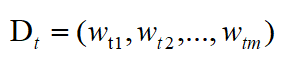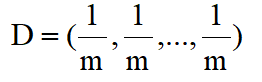2）定义第 t 轮弱学习器的误差

(还有另外一种，计算准确率，不过这样的话后面4）对样本权重的计算就改反了)

（也就是说，学习器如这里的CART，使用的时候还是不需要管其样本权重这个集成里面的概念的，其单独训练完成以后，样本权重最终使用的地方就在交叉验证等计算错误率的时候，乘上了权重，就是我们的弱学习器的误差了

3）定义第 t 轮学习器其本身的权重

（怎么来的后面解释推导）

（可见，正确率/误差率，越小的权重越大）

（可见，这里的时候，如0.6：，是非法的，此时要么终止，要么重头/重新学习一个

4）定义第 t轮学习器，权重分布D的更新方式

（怎么来的后面解释推导）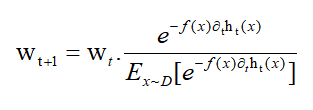(如果2）是基于计算准确率的，那么这里就要反一下，比如去掉里面的负号，那么分错的样本权重越小，对的越大)

5）最终的强学习器

Adaboost分类采用的是加权表决法，按照4.1那T轮，根据上面1)-4)的计算后，最终的强分类器为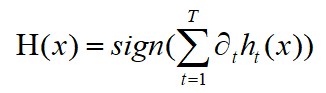6）对3）、4）的推导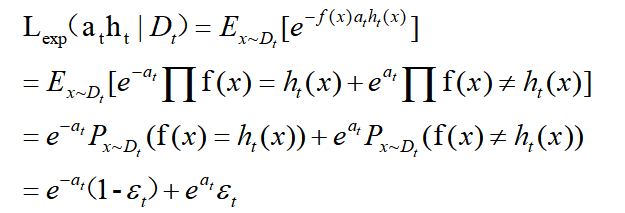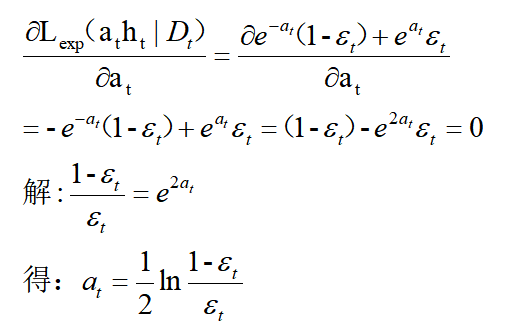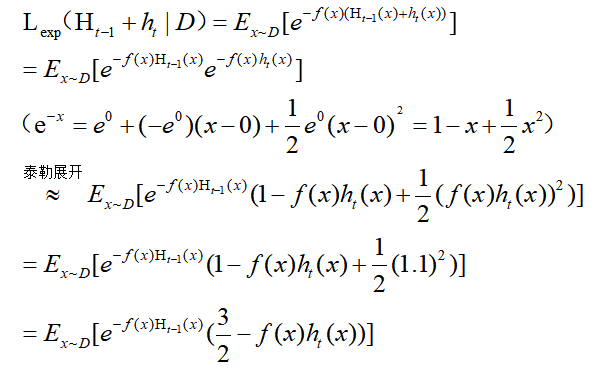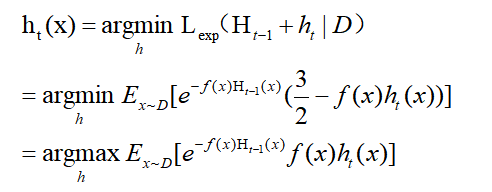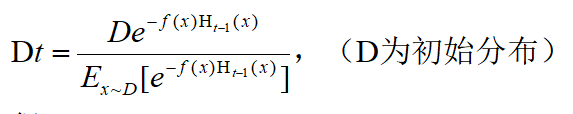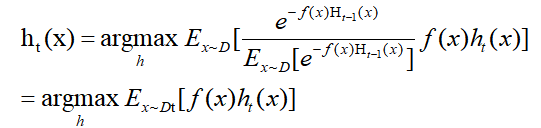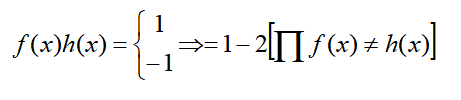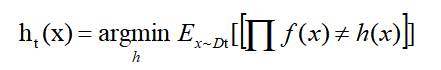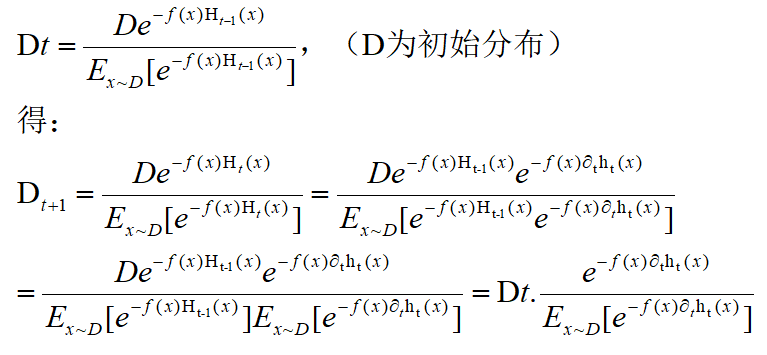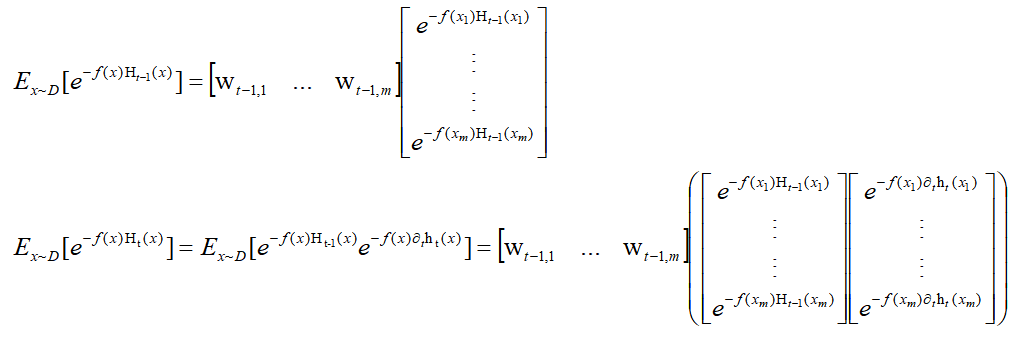7）最终Adaboost分类流程

1）设置分布D0=1/m

2）for t=1,2,....,T（T个弱学习器，这个自己指定） do:

ht=Algri(D,Dt)    //根据Dt样本权重分布，从数据集D中训练出弱模型，如CART

epsilon_t=P(ht(x)!=f(x))   //上面的2)节形式计算第t轮弱学习器误差

if epsilon_t>0.5 then break  //训练太差就不进一步学了，这样实际上得不到T个弱学习去，因此这种情况时，还可以从初始分布或者随机分布中学到ht。

按照上面 3）节更新

对分布Dt 中每个wti更新，按照4）节形式更新

3）end for

4) 按照5）节中形式加权累积起来作为集成模型输出。

from sklearn.model_selection import KFold
from sklearn.model_selection import cross_val_score

from sklearn.ensemble import AdaBoostClassifier
from sklearn.tree import DecisionTreeClassifier

from sklearn import datasets

dataset = datasets.load_breast_cancer()
X = dataset.data
Y = dataset.target

seed = 1

# 10折交叉验证
kfold = KFold(n_splits=10, random_state=seed)
# 弱分类器：CART(criterion='gini'表示用CART),设置弱分类器maxdepth=3
dtree = DecisionTreeClassifier(criterion='gini', max_depth=3)

# dtree = dtree.fit(X, Y)
# result = cross_val_score(dtree, X, Y, cv=kfold)
# print(result.mean())

model = AdaBoostClassifier(base_estimator=dtree, n_estimators=100, random_state=seed)
result = cross_val_score(model, X, Y, cv=kfold)

print('10折交叉验证的分数：',result.mean())

#10折交叉验证的分数： 0.9666040100250626


### 4.2.2.回归

1）定义第 t 个弱学习器，训练集上的最大误差

2）样本的误差

（相对误差）、（平方误差）

（指数误差）

3）第 t 个弱学习器的误差

4）第t个学习器权重

（可见这里是错误率越高， 权重越大。）

5）第 t 个弱学习器的第 i 个样本权重更新

6）最终集成学习器为

（可见，越大，越小，此时作用在hc上的权值越小，而4）说了，错误率越大越大，因此，错误率越大的作用在hc上越小）

from sklearn.model_selection import KFold
from sklearn.model_selection import train_test_split
import numpy as np
from sklearn.ensemble import AdaBoostRegressor
from sklearn.tree import DecisionTreeRegressor
from sklearn.svm import LinearSVR
from matplotlib import pyplot as plt

from mpl_toolkits.mplot3d import Axes3D

fig = plt.figure()
plt.rcParams['font.sans-serif'] = ['SimHei']  # 用来正常显示中文标签
plt.rcParams['axes.unicode_minus'] = False  # 用来正常显示负号
ax = Axes3D(fig)

seed = 1

def load_data_regression():
# 构造X数据
c=np.random.rand(220)
X =np.array([c+2*i for i in range(2)]).T
# 构造y数据， y = 1 * x_0 + 2 * x_1 + 3，后面打印参数会发现，是一致的
y = np.dot(X, np.array([1, 2])) + 1

return train_test_split(X, y, test_size=0.2, random_state=0)

X_train, X_test, y_train, y_test = load_data_regression()
# 10折交叉验证
kfold = KFold(n_splits=10, random_state=seed)
# 弱回归器：CART(criterion='gini'表示用CART),设置弱回归器maxdepth=3
dtree = DecisionTreeRegressor(max_depth=3)

# model = AdaBoostRegressor(base_estimator=LinearSVR(epsilon=0.01, C=100), n_estimators=200, random_state=seed)
model = AdaBoostRegressor(base_estimator=dtree, n_estimators=300, random_state=seed)
model.fit(X_train, y_train)

result = model.predict(X_test)
# # 计算准确率
cnt1 = 0
cnt2 = 0
for i in range(len(y_test)):
if abs(result[i] - y_test[i]) < 0.1:
cnt1 += 1
else:
cnt2 += 1

print("Accuracy: %.2f %% " % (100 * cnt1 / (cnt1 + cnt2)))

ax.scatter(X_train[:, 0], X_train[:, 1], y_train, marker='o')
ax.plot(X_test[:, 0], X_test[:, 1], result)
plt.show()


### 4.2.4Adaboost小结

这里对Adaboost算法的优缺点做一个总结。

Adaboost的主要优点有：

1）Adaboost作为分类器时，分类精度很高

2）在Adaboost的框架下，可以使用各种回归分类模型来构建弱学习器，非常灵活。

3）作为简单的二元分类器时，构造简单，结果可理解。

4）不容易发生过拟合

Adaboost的主要缺点有：

1）对异常样本敏感，异常样本在迭代中可能会获得较高的权重，影响最终的强学习器的预测准确性。

# 5.Bagging

Bagging算法是另外一类，可以并行计算，不像前面的AdaBoost，是一个依赖一个的串行的。这种集成算法数学性并不要求很高。简单易理解。

# 5.1基本流程

1）基于自助采样给定m个数据，有放回的采样m次，得到一个包含m个数据的数据集（有些会出现多次，有些不出现，但理论上只有63.2%的概率出现在集合中）。

2）自助采样T次，就得到了T个含m个数据的数据集。

3）T次采样每次采样完都训练出一个弱学习器，这样得到T个弱学习器了。

4）预测，对分类任务：使用投票多数表决了；回归：简单平均。

# 6.随机深林Random Forest

（值得说明的是，随机森林通常优于Bagging，因为显然二者都是基于随机的，但是随机森林随机的更加彻底）

## 6.1基本流程

1）按照Bagging那样随机自助采样得到m个数据集

2）运用决策树算法，利用采样数据进行弱学习器学习，但是决策树每次划分的时候是在当且可选属性d中挑选k个(k<=d)然后再选择最优属性划分。

3）重复采样、学习T次，得到T个弱学习器，

4）和Bagging一样，对分类任务：使用投票多数表决了；回归：简单平均。

from sklearn.model_selection import cross_val_score
from sklearn.datasets import make_blobs
import matplotlib.pyplot as plt
from sklearn.ensemble import RandomForestClassifier
from sklearn.ensemble import ExtraTreesClassifier

from sklearn.tree import DecisionTreeClassifier

seed = 1
X, y = make_blobs(n_samples=1000, n_features=6, centers=50, random_state=seed)

# 普通决策树
clf = DecisionTreeClassifier(max_depth=None, min_samples_split=2, random_state=seed)
scores = cross_val_score(clf, X, y)
print(scores.mean())

# 随机森林
clf = RandomForestClassifier(n_estimators=10, max_depth=None, min_samples_split=2, random_state=seed)
scores = cross_val_score(clf, X, y)
print(scores.mean())

# RF随机森林的变种
clf = ExtraTreesClassifier(n_estimators=10, max_depth=None, min_samples_split=2, random_state=seed)
scores = cross_val_score(clf, X, y)
print(scores.mean())


# 7.Stacking

stacking产生方法是一种截然不同的组合多个模型的方法，它不像bagging和boosting是同质组合，而是组合不同的模型，具体的过程如下：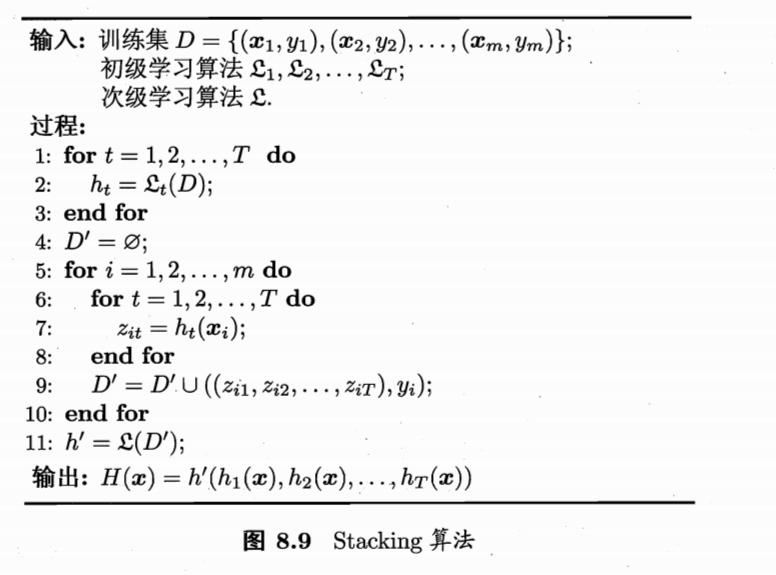（过程7：每个数据xi经过T个初级学习器预测之后，会输出T个值，zi与xi的真正值yi组成新的数据D'=(zi,yi)，这个数据会被用来在次级学习器中进行学习，产生m个这样的D'，组成了次级学习器的训练集。

from sklearn import model_selection
from sklearn.linear_model import LogisticRegression
from sklearn.neighbors import KNeighborsClassifier
from sklearn.naive_bayes import GaussianNB
from sklearn.ensemble import RandomForestClassifier
from mlxtend.classifier import StackingClassifier
import numpy as np

# 初级学习器
clf1 = KNeighborsClassifier(n_neighbors=1)
clf2 = RandomForestClassifier(random_state=1)
clf3 = GaussianNB()

# 次级学习器
lr = LogisticRegression()

# sclf = StackingClassifier(classifiers=[clf1, clf2, clf3],
#                           meta_classifier=lr)

#use_probas=True使用初级学习器的概率作为输入，没有的话直接使用其输出作为输入。
# average_probas=False，不使用初级的平均作为输入,拼接起来作为输入。
'''
classifier 1: [0.2, 0.5, 0.3]

classifier 2: [0.3, 0.4, 0.4]

1) average = True :

2) average = False:

'''
sclf = StackingClassifier(classifiers=[clf1, clf2, clf3],
use_probas=True,
average_probas=False,
meta_classifier=lr)
# 3折交叉验证
print('3-fold cross validation:\n')

from sklearn import datasets

from sklearn import datasets

from sklearn.datasets.samples_generator import make_classification

# X为样本特征，Y为样本类别输出， 共1000个样本，每个样本2个特征，输出有3个类别，没有冗余特征，每个类别一个簇
X, y = make_classification(n_samples=1000, n_features=2, n_redundant=0,
n_clusters_per_class=1, n_classes=3)

for clf, label in zip([clf1, clf2, clf3, sclf],
['KNN',
'Random Forest',
'Naive Bayes',
'StackingClassifier']):
scores = model_selection.cross_val_score(clf, X, y,
cv=3, scoring='accuracy')
print("Accuracy: %0.2f (+/- %0.2f) [%s]"
% (scores.mean(), scores.std(), label))

#
3-fold cross validation:

Accuracy: 0.96 (+/- 0.00) [KNN]
Accuracy: 0.97 (+/- 0.00) [Random Forest]
Accuracy: 0.95 (+/- 0.01) [Naive Bayes]
Accuracy: 0.97 (+/- 0.00) [StackingClassifier]

# 8.GBDT

GBDT也是迭代型的，可以用于分类和回归，弱学习器限定只能使用CART回归树模型，要注意的是这里的决策树是回归树，GBDT中的决策树是个弱模型，深度较小一般不会超过5，叶子节点的数量也不会超过10

## 8.1 GBDT回归

1）假设前一轮学到的强学习器是：

2）定义损失为：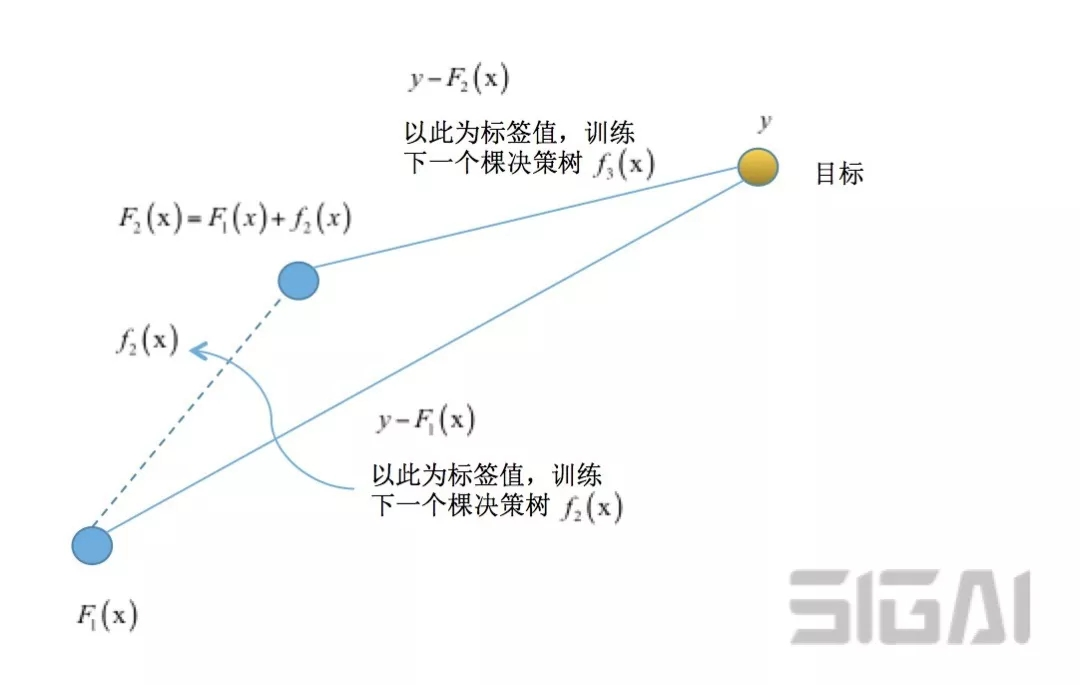L可以这么选，GBDT通常是平方损失，也就是下1，因此对于，其负梯度损失可以看作是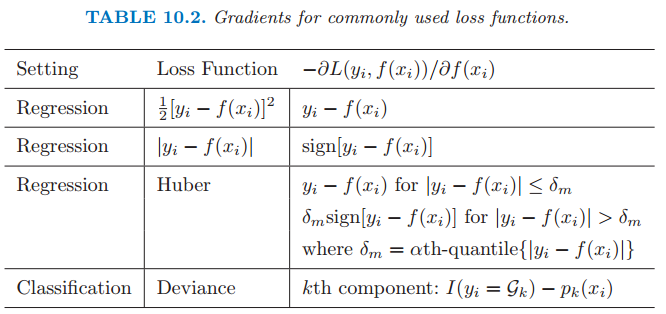3）构造出CART树

a）计算出每个样本的损失负梯度值（是个值），得到新的数据集（自变量及对应损失梯度值构成的集合）：，就可以可以开始构造CART回归树了。

b)记叶子节点为：，J为叶子节点个数。假设叶子节点包含k个样本，我们希望这个叶子节点的输出能让节点内k个样的累积损失最小（因为每个节点都这样计算最佳c，整个树的最佳输出就找到了）：

c)根据CART构造规则，叶子节点的值为节点内数据的平均或中位数。但是现在叶子节点内部k个样本负梯度的均值记avgrtj是梯度均值。（问题是梯度只是个方向，梯度=0.1=100=无穷 >0，仅仅表示下一轮弱学习器应该往增大预测输出这个方向上去）（有些资料直接拿这个做输出也是可以的，就是学习率是1，然后执行了一次梯度下降嘛。但是不一定就是最佳输出，可以用一维线性搜索：https://blog.csdn.net/jiang425776024/article/details/87600422比如设置学习率因子为0.1，然后利用节点的那个平均负梯度值为梯度，直到n步后损失值收敛或不下降，这时累积找到的作为最佳输出

d)划分CART树就是正常那样方差最小划分，节点输出为基于节点负梯度均值为梯度下降方向，找到的最佳c为此节点的输出，这样，就能构造出新的CART树了。

4）得到CART决策树函数

5）得到新的强学习器

6）得到最终集成器

## 8.2GBDT分类

### 二分类

GBDT的分类算法从思想上和GBDT的回归算法没有区别，但是由于样本输出不是连续的值，而是离散的类别，导致我们无法直接从输出类别去拟合类别输出的误差。

1）这里记分类标签为-1,1，定义损失形式为：

（负二项对数似然）

2）然后就能计算负梯度函数了：

3）叶子节点估计值：

4）这样，基于CART划分节点规则，基于上面对节点的输出，可以构造出CART回归树。

5）其它步骤与上面的回归一致。

6）最后预测形式

### 多分类

1）定义损失

，yk∈0,1.

2）定义概率p

Softmax数值转化为相对概率，显然，初始时pk=第k类样本的占比，因为 fl(x)=0，e^0=1。

3）xi第 t 轮的第k类 的负梯度误差

(多分类假设有K=5个类，已知xi是1类，那么yi=[0,1,0,0,0]，yi1=1,其余为0，也就是说，每个样本要对K个类都计算出概率)

4）x的第t轮第j个叶子节点的第l类输出

（每个数据xi会划分到K棵树的K个不同的叶子节点内(j不一样)，有K个不同的损失负梯度值，对应于xi的K个类的残差）

5）x的第 k类的第 t 轮输出

6）得到x的最终预测

T轮学完后，第k个类的输出函数为

# 9.XGBoost

Xgboost是GB算法的高效实现，xgboost中的基学习器除了可以是CART（gbtree）也可以是线性分类器（gblinear）。

xgboost在目标函数中显示的加上了正则化项，正则化项与树的叶子节点的数量T和叶子节点的值有关。

## 1）定义结构函数q(x)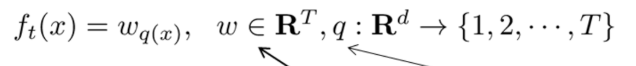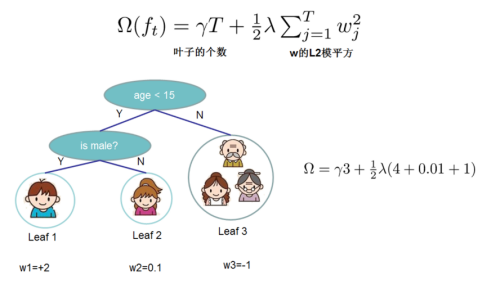## 2）输出函数的叠加形式为：## 3）第 t 轮的目标（损失）函数为：Logistic损失：如果我们考虑使用平方误差作为损失函数，公式可改写为：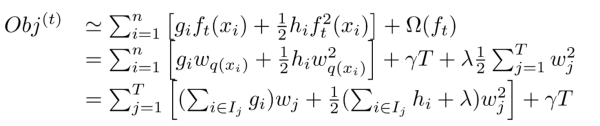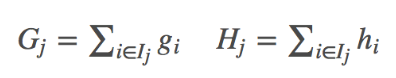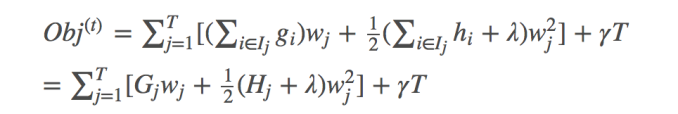## 4）结构评价函数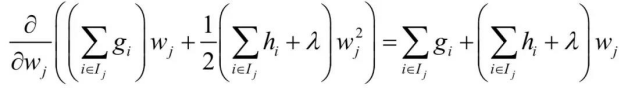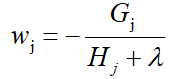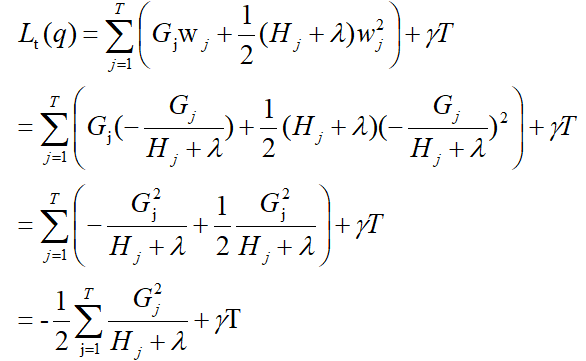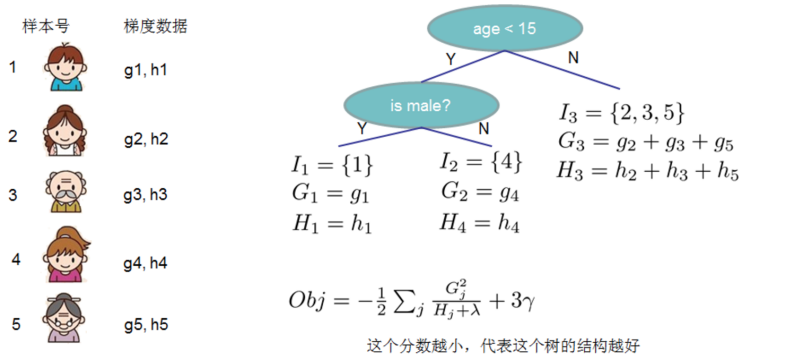## 5）确定最佳分裂准则

XGBoost是一层层往下分的，先简单来说，最初只有根节点，1个叶子节点，包含整个的训练集样本，好了，这个时候怎么分裂为二变成3个节点，两个叶子节点呢？这个过程清楚了，其它所有节点都这样递归下去就行了。

a）原始树q是这样子，给定损失函数就能计算出一二阶导数，进而求得G，H，给定了，那么这个树q的分数就可以唯一确定了，计算即可。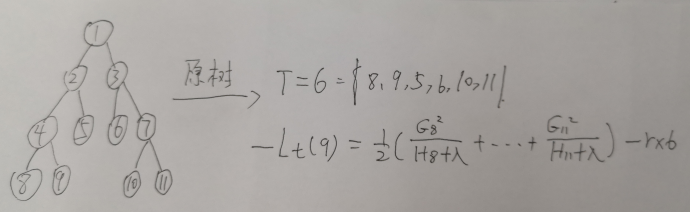b）假设现在我们要对节点9进行分裂，假设我们通过某种划分把9中的数据划分成左右2个部分9L/9R了，记为节点12,13（划分方式，怎么划分为两个数据集，参考CART树的划分方式）。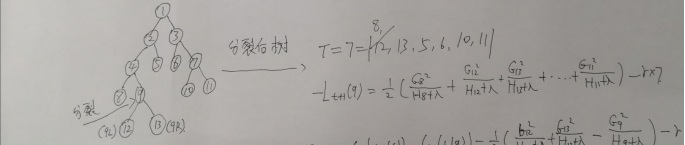c）那么现在我要比较两个树的分数了，减法嘛，小学生都知道，我们记减法后的结果为Gain，显然这是个数(Gain>0意味着划分后的结构分数更高，Gain=0意味着划分不划分都一样，Gain<0意味着划分后变差了)：

（请详细看最后那步的结果，最终两颗树分数的相减结果，只与划分点9有关，这也很符合常识，因为这两颗树仅仅在9那里有区别，最后一步的第3项之所以能这样展开，是因为显然有这样的关系：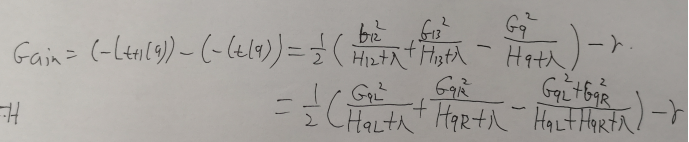d）调参阀门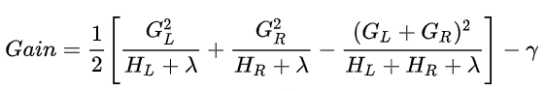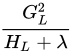代表左子树分数，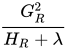代表右子树分数，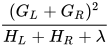代表不分割时我们可以获得的分数，为新加入叶子节点引入的复杂度代价。

## 6）分裂流程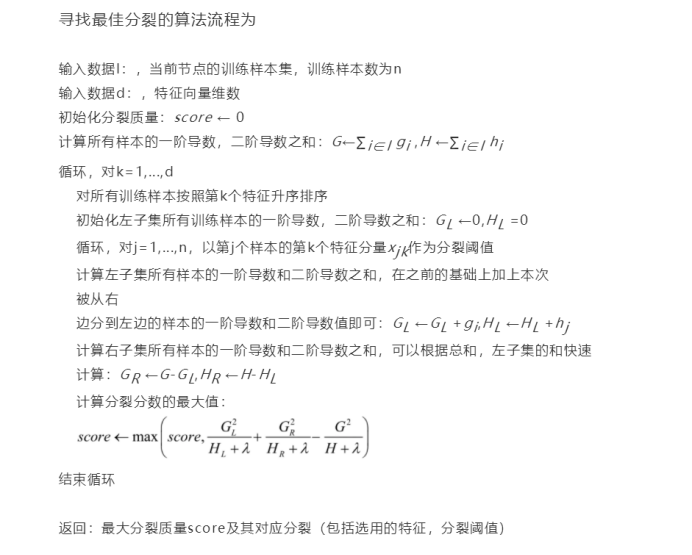xgboost考虑了训练数据为稀疏值的情况，可以为缺失值或者指定的值指定分支的默认方向，这能大大提升算法的效率，paper提到50倍。

xgboost 还考虑了当数据量比较大，内存不够时怎么有效的使用磁盘，主要是结合多线程、数据压缩、分片的方法，尽可能的提高算法的效率。

import numpy as np
import xgboost as xgb
from xgboost import plot_importance
from matplotlib import pyplot as plt
from mpl_toolkits.mplot3d import Axes3D
from sklearn.model_selection import train_test_split

fig = plt.figure()
plt.rcParams['font.sans-serif'] = ['SimHei']  # 用来正常显示中文标签
plt.rcParams['axes.unicode_minus'] = False  # 用来正常显示负号
ax = Axes3D(fig)

# read in the iris data
# 构造X数据
X = np.random.randint(0, 7, (2000, 2))
# 构造y数据， y = 1 * x_0 + 2 * x_1 + 3，后面打印参数会发现，是一致的
y = np.dot(X, np.array([1, 2])) + 3

X_train, X_test, y_train, y_test = train_test_split(X, y, test_size=0.2, random_state=0)

# 训练模型
model = xgb.XGBRegressor(max_depth=5, learning_rate=0.1, n_estimators=100, silent=True, objective='reg:linear')
model.fit(X_train, y_train)

# 对测试集进行预测
ans = model.predict(X_test)
print(ans)
ax.scatter(X_train[:, 0], X_train[:, 1], y_train, marker='o')
ax.plot(X_test[:, 0], X_test[:, 1], ans)

# # 计算准确率
cnt1 = 0
cnt2 = 0
for i in range(len(y_test)):
if abs(ans[i] - y_test[i])<0.1:
cnt1 += 1
else:
cnt2 += 1

print("Accuracy: %.2f %% " % (100 * cnt1 / (cnt1 + cnt2)))

# 显示重要特征
plot_importance(model)
plt.show()


分类使用：

from sklearn.datasets import load_iris
import xgboost as xgb
from xgboost import plot_importance
from matplotlib import pyplot as plt
from sklearn.model_selection import train_test_split

# read in the iris data
iris = load_iris()

X = iris.data
y = iris.target

X_train, X_test, y_train, y_test = train_test_split(X, y, test_size=0.2, random_state=0)

# 训练模型
model = xgb.XGBClassifier(max_depth=5, learning_rate=0.1, n_estimators=160, silent=True, objective='reg:logistic')
model.fit(X_train, y_train)

# 对测试集进行预测
ans = model.predict(X_test)

# 计算准确率
cnt1 = 0
cnt2 = 0
for i in range(len(y_test)):
if ans[i] == y_test[i]:
cnt1 += 1
else:
cnt2 += 1

print("Accuracy: %.2f %% " % (100 * cnt1 / (cnt1 + cnt2)))

# 显示重要特征
plot_importance(model)
plt.show()

# 10.LightGBM

LightGBM是个快速的，分布式的，高性能的基于决策树算法的梯度提升框架。可用于排序，分类，回归以及很多其他的机器学习任务中。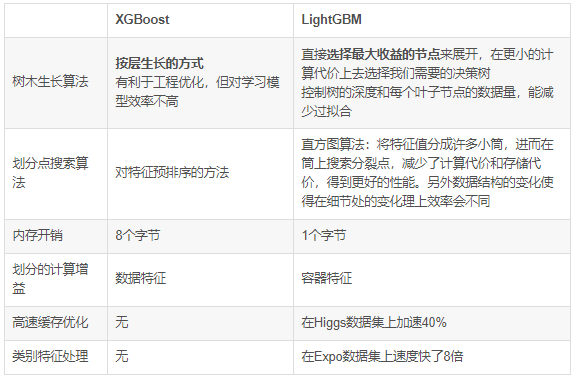LightGBM的改进更多是工程上的改进，可能准确率并不会比XGBoost高，但是速度快很多。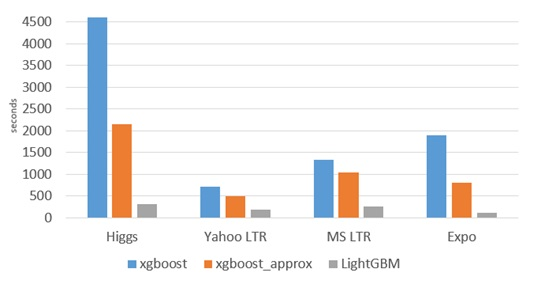1、安装Anaconda3

2、pip install lightgbm

LightGBM 的所有参数介绍：http://lightgbm.apachecn.org/#/docs/6

# 11.CatBoost

Yandex 的 CatBoost 号称是比 XGBoost 和 LightGBM 在算法准确率等方面表现更为优秀的算法。